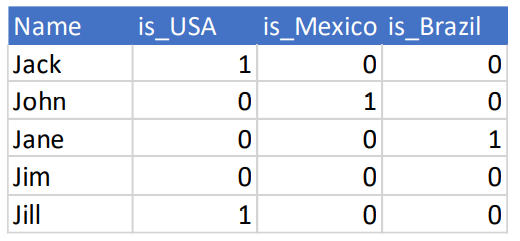top of page
Search

# Feature Engineering And Linear Regression In Machine Learning

### Machine Learning Pipeline• The initial process in any machine learning implementation

• The purpose is to understand the data, interpret the hidden information, visualizing and engineering the feature to be used by the machine learning

• A few things to consider:

– What questions do you want to answer or prove true/wrong?

– What kind of data do you have? Numeric, Categorical, Text, Image?

How are you going to treat them.

– Do you have any missing values, wrong format, etc.

– How the data is spread? Do you have any outliers? How are you going to

deal with them?

– Which features are important? Can we add or remove features to get

more from the data?

### Data Wrangling

– Understand the data

– Getting basic summary statistics

– Handling missing values

– Handling outliers

– Typecasting and transformation

### Data Visualization

– Univariate Analysis: histogram, distribution (distplot, boxplot, violin)

– Multivariate Analysis: scatter plot, pair plot, etc

### Feature Engineering

• Why Feature Engineering?

– Better representation of data

– Better performing models

– Essential for model building and evaluation

– More flexibility on data types

– Emphasis on the business and domain

• Types of data for feature engineering ranges from numerical, categorical, text, temporal, and image

### Numerical data

• Can be used in raw

• Rounding

• Counts of numeric data, e.g., frequency of songs listened by users

• Binarization, e.g., instead of frequency we can put a ‘0’ or ‘1’ value to state whether a song has been listened by a user (for a recommender system)

• Binning, e.g., categorize users based on some age groups

• Interaction or combination, for example by using polynomial features

• Transformation, e.g., log transform, polynomial transform

### Categorical data

• Transform the data into nominal feature, e.g., for a movie genre, you can have {0: ‘action’, 1: ‘thriller’, 2: ‘drama’, 3: ‘horror’, 4: ‘comedy’, 5: ‘family’, 6: ‘other’}

• Transform into ordinal value, e.g., similar to the above, but there is an order in which the category or genre is introduce in the data

### Encoding:

– Use dummy encoding

– Transform a categorical feature of m distinct labels into m-1 binary features

### Categorical data

Consider the following dataframe:After the dummy encoding scheme:If we drop the first (is_USA) or the last (is_Canada), will it destroy the dataset?### Feature Scaling

• Using the features’ raw value might make models biased towards features with really high magnitude

– Outliers will skew the algorithm

– Affects machine learning algorithms that use the magnitude of features,

i.e., regression

• Scikit-learn’s preprocessing module provide three different feature scaler: standard scaler, minmax scaler and robust scaler

• Standard scaler (aka Z-score scaling) tries to remove the mean and scale the variance into 1MinMax scaler scale the feature value in range [0 1] by utilizing its minimum and maximum values– Be careful with outliers in minmax scaler

Robust scaler using some statistical measures like median and percentile to scale the data– IQR is Inter-Quartile Range is the range or differences between the 75% quartile and 25% quartile

Filter Methods

– It is based on metrics like correlation, features’ values, and does not

depends on results from any model

– Popular methods are threshold and statistical method

Wrapper Methods

– Use recursive approach to build multiple models using feature subsets

to select the best subsets. The RFE (Recursive Feature Elimination)

from sklearn.feature_selection is one such example

– Popular methods are ANOVA and chi-square tests – Utilize regression/classifier and cross-validation

Embedded Methods

– Use machine learning algorithms like random forests, decision trees and ensemble methods to rank and score features

Threshold Methods

– You can analyze features’ variant

– Features that are less variant, i.e., mostly constant across all observation can be removed### Dimensionality Reduction

• Dealing with a lot of features can lead to issues like model overfitting, complex models, and many more that all roll up to what is called as the curse of dimensionality

• Dimensionality reduction is the process of reducing the total number of features in our feature set using strategies like feature selection or feature extraction.

• A very popular technique for dimensionality reduction is called PCA (principal component analysis)

REGRESSIONRegression XP

• We build regression models to explain and to predict phenomena

Regression Model

– Continuous (Multiple Regression)

• Linear and non-linear

– Discrete (Logistic Regression)

• Binary and multinomial

Regression analysis attempts to explain the influence that input (independent) variables have on the outcome (dependent) variable

Linear Multiple Regression

Models the relationship between some input variables and a continuous outcome variable

– Assumption is that the relationship is linear

– Transformations can be used to achieve a linear relationship.

Remember the transformation we did to the dataframeMultiple Linear Regression Use Case

Real estate example

– Predict residential home prices

• Possible inputs – living area, #bathrooms, #bedrooms, lot size, property taxes

Demand forecasting example

– Restaurant predicts quantity of food needed

• Possible inputs – weather, day of week, etc.

Medical example

– Analyze effect of proposed radiation treatment

• Possible inputs – radiation treatment duration, frequeny